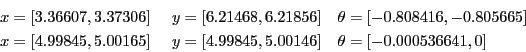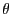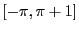Next: Example 3 Up: Examples Previous: Example 1   Contents

Example 2

The problem we want to solve has been presented in section 2.3.5.2,15.1.1.

INTERVAL_MATRIX IntervalGradient (int l1,int l2,INTERVAL_VECTOR & in)
{
INTERVAL x,y,teta;
x=in(1);y=in(2);teta=in(3);
if(l1==1)
{
}
if(l1==2)
{
}
if(l1==3)
{
if(l2==3){
4.0*Sin(teta))*y+(-4.0*Sin(teta)-4.0*Cos(teta))*x;
}
}
}
We may use the same main program as in the previous example (the name of this program is

Let's assume that we set epsilonf to 0 and epsilon to 0.01 while looking at all the solutions (Stop=0), using the maximum equation ordering and setting Dist to 0.1. The algorithm provide the following solutions after using 55 boxes:We notice that all of the roots are contained in the solution intervals.

If we use the maximum middle-point equation ordering the algorithm provide the same solution intervals.

With epsilonf=0.001, epsilon=0 the algorithm still find exactly the root with 55 boxes and a computation time of 7010ms. Here Moore test may have failed as the solution inis 0, which correspond exactly to split point in the bisection process: it may be useful to break the symmetry in the test domain.

Using the single bisection mode and setting the flag Single_Bisection to 2 enable to reduce the number of boxes to 33 and the computation time to 3580ms for epsilonf=0.00001.

Note that we may improve the efficiency of the procedure by using simplification procedures such as the 2B (section 2.17) and the 3B method. In that case for epsilonf=1e-6, epsilon=1e-6 the number of boxes will have been reduced to 7. Note that the solution [5,5,0] is still not guaranteed. But using a search space offorallow the Moore test to guarantee both solutions.Next: Example 3 Up: Examples Previous: Example 1   Contents
Jean-Pierre Merlet 2012-12-20